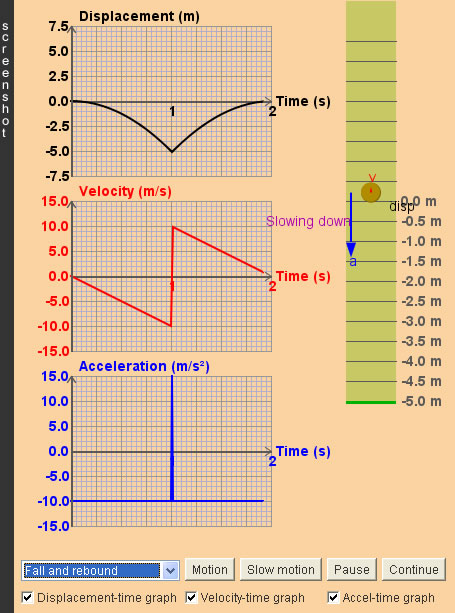Kinematics of 1-D Uniformly Accelerated Motions

1. Here, 'displacement' refers to that from the starting position.
2. When 'Edit v-t graph' is selected, the v-t graph is capable to be changed by dragging the red corner points on the graph upward or downward.
3. The slope of displacement-time graph is velocity; the slope of velocity-time graph is acceleration. Uniform acceleration is the case when the acceleration is a constant,i.e. the velocity changes at a constant rate.
4. Negative acceleration is usually miscalled "deceleration". In fact, we need both the directions of velocity and acceleration to determine whether the object is speeding up or slowing down. The following table summarizes the four cases:
 Velocity Acceleration Status Positive Positive Real acceleration (moving in the positive direction and speeding up) Positive Negative Real deceleration (moving in the positive direction and slowing down) Negative Positive Real deceleration (moving in the negative direction and slowing down) Negative Negative Real acceleration(moving in the negative direction and speeding up) In short, an object slows down when its acceleration is opposite to its velocity.

 Internal Links : Newton's first law of motion Applying force to object Spring-Mass oscillator (Kinematics)

 The author (Chiu-king Ng) has the copyright on all the simulations in this website. Email phyAA@phy.hk, where AA is the prime number following 7. Last Update:2019-9-15